Published: 28 June 2021

# A new feature of magnetic memory signal for quantitative identification of stress concentration

Shujun Liu1
Qiwei Yong2
Dean He3
Yonggang Zuo4
Zhen Zhang5
Guodong Zeng6
1, 2, 3, 4, 5, 6Army Logistics Academy, Chongqing, China
Corresponding Author:
Shujun Liu
Views 48

#### Abstract

Magnetic memory detection technology is a new nondestructive testing technology developed in recent 20 years, which can detect the stress concentration of ferromagnetic materials. At present, the quantification of stress concentration in magnetic memory testing is always a difficult problem. In the quantitative research of magnetic memory detection, the key is to extract the effective characteristics of magnetic memory signal. In this paper, a feature of magnetic memory signal based on wavelet packet energy spectrum is proposed. The experimental results show that the stress concentration of ferromagnetic materials is related to the wavelet packet energy spectrum distribution of magnetic memory signals. The lower the stress concentration, the more scattered the energy spectrum distribution of wavelet packet. The higher the stress concentration is, the more concentrated the energy spectrum of wavelet packet is, and the energy of wavelet packet is mainly concentrated in the low frequency band.

## 1. Introduction

Nondestructive testing (NDT) is a technology to detect the defects, chemical and physical parameters of materials, parts and equipment without damaging or affecting the performance of the tested object. Nondestructive testing technology plays an important role in industry. The common nondestructive testing technologies include ultrasonic testing, radiographic testing, eddy current testing, magnetic particle testing, etc. However, the traditional nondestructive testing technology can only detect macro defects, and cannot do anything about the early damage of materials, parts and equipment such as stress concentration. Magnetic memory testing (MMT) is a new non-destructive testing technology in recent years. It is a rapid non-destructive testing method which uses metal magnetic memory effect to detect the stress concentration of components. It overcomes the shortcomings of traditional nondestructive testing and can diagnose the internal stress concentration area of ferromagnetic metal components, namely micro defects and early failure and damage, so as to prevent sudden fatigue damage.

## 2. Basic principle of magnetic memory testing

During the processing and operation of ferromagnetic metal parts, due to the combined action of load and geomagnetic field, the magnetostrictive orientation and irreversible reorientation of magnetic domain structure will occur in the stress and deformation concentration area. The irreversible change of the magnetic state is not only retained after the working load is removed, but also related to the maximum applied stress. This kind of magnetic state on the surface of metal component memorizes the location of micro defect or stress concentration, which is called magnetic memory effect. As shown in Fig. 1, when the ferromagnetic component in the geomagnetic field is subjected to external load, the magnetostrictive orientation and irreversible reorientation of the magnetic domain structure will occur in the stress concentration region. The fixed nodes of the domain will appear in this part, and the magnetic poles will be generated to form the demagnetic field. Thus, the permeability of ferromagnetic metal is minimum, and the leakage magnetic field is formed on the metal surface. The tangential component $Hp\left(x\right)$ of the MFL intensity has the maximum value, while the normal component $Hp\left(y\right)$ changes sign and has zero value. This irreversible change of magnetic state still retains and memorizes the stress concentration position after the working load is removed. Based on the basic principle of metal magnetic memory effect, the instrument can evaluate the stress concentration and the existence of micro defects by recording the distribution of the magnetic field intensity component perpendicular to the surface of the metal component along a certain direction [1-3].

Fig. 1Schematic diagram of metal magnetic memory testing principle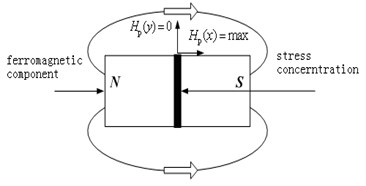## 3. Difficulties in quantitative detection of magnetic memory

In the early diagnosis of ferromagnetic metal materials, magnetic memory testing technology is the only feasible nondestructive testing method so far. Its birth to a certain extent makes up for the shortcomings of traditional nondestructive testing technology, and has become a research hotspot in the field of nondestructive testing. However, there is a complex nonlinear relationship between stress concentration and magnetic memory signal. The current criterion of stress concentration in magnetic memory detection can only be used to determine the stress concentration position, and it is not possible to further accurately identify the degree of stress concentration. This greatly hinders the development and application of magnetic memory testing. At present, researchers mainly use experiments to study the quantitative identification of early damage or defect of ferromagnetic materials. They used uniaxial and biaxial tensile tests or fatigue tests under various typical loads to analyze the qualitative relationship between the average stress and the magnetic memory signal, and fitted the quantitative relationship between the magnetic field signal and the stress from a macro perspective through a large number of experimental statistics. But this kind of experiment can’t reflect the real size of stress, especially the real situation of stress concentration [4, 5]. In fact, in the problem of quantifying stress concentration using magnetic memory, extracting effective features of magnetic memory signal is the key. Only by extracting the magnetic memory signal which can represent the stress concentration mode information, can the quantification of the stress concentration be realized.

### 3.1. Wavelet packet energy spectrum of magnetic memory signal

Wavelet packet decomposition is a more precise orthogonal decomposition method based on multi-resolution wavelet transform. It can decompose the signal in the whole band, and get more precise and comprehensive information. Therefore, it has better adaptability and is easy to extract the characteristic frequency from the signal. Wavelet packet decomposition is widely used in signal frequency decomposition. Therefore, this paper intends to use the wavelet packet decomposition method to decompose the magnetic memory in the whole frequency band, so as to realize the feature extraction of stress concentration in the frequency range of the signal [6, 7].

Wavelet packet decomposition can decompose signals into different frequency bands without leakage and overlap according to any time-frequency resolution. After wavelet packet transform, the information is complete. All frequencies are reserved, which provides a powerful condition for extracting the main information from the signal. This kind of decomposition can be carried out as many times as needed to obtain the required frequency. Assuming that the wavelet packet decomposition level of signal $S$ is $j$ level, the signal can be decomposed into $j$ level and ${2}^{j}$ nodes. Assuming that the series of wavelet packet decomposition coefficients of a certain frequency band is $\left\{{x}_{k}\right\}$, the wavelet packet energy of the frequency band is given by Eq. (1):

1
${E}_{jm}=\sum _{k=1}^{n}\left({x}_{{\mathrm{}}^{k}}{\right)}^{2}.$

When the energy elements in each frequency band of the $j$ layer are combined into an eigenvector $\left[{E}_{j0},{E}_{j1},\cdots ,{E}_{j{2}^{j}-1}\right]$, the eigenvector $\left[{E}_{j0},{E}_{j1},\cdots ,{E}_{j{2}^{j}-1}\right]$ is called the wavelet packet energy spectrum of the signal $S$, which is called $\left[{E}_{jm}\right]$. The total energy of the signal in each frequency band is consistent with that of the original signal. The magnetic memory signal in each frequency band represents the characteristic information of the original signal in the frequency range. The distribution of stress concentration has a great influence on the energy in each frequency band. When the energy is large, $\left[{E}_{jm}\right]$ is usually a relatively large value. It will bring some inconvenience in data analysis. In order to make the energy distribution of different magnetic memory signals comparable, it is necessary to normalize the wavelet packet energy spectrum. By normalizing $\left[{E}_{jm}\right]$, the normalized wavelet packet energy spectrum $\left[{E}_{jm}/E\right]$ of the signal $S$ is obtained. The total energy of the signal $E$ is given by Eq. (2):

2
$E=\sqrt{\sum _{m=0}^{{2}^{j}-1}{E}_{jm}}.$

It can be seen that wavelet packet decomposition decomposes the frequency band many times. It can re decompose the high frequency part without subdivision in wavelet decomposition, and adaptively select the corresponding sub-band according to the characteristics of the signal to be decomposed, so that the sub-band matches the spectrum of the signal. After wavelet packet decomposition, all the feature information of the signal can be preserved, which provides strong support for extracting the feature information in the signal. If there are too many decomposition levels, the dimension of the data to be processed will be increased. In practical application, we need to choose an appropriate decomposition level according to the actual situation. After analyzing and testing the magnetic memory signal data, we can find that it is the most appropriate to decompose it into three layers.

## 4. Experiment

In order to study the wavelet packet energy spectrum of magnetic memory signal, the following experiments are carried out. The magnetic memory signal is collected and analyzed by wavelet packet.

### 4.1. Experimental equipment and materials

The experimental instruments are MFL -4032 MFL / MMM detector and MTS810 hydraulic servo testing machine, as shown in Fig. 2 and Fig. 3. The static load error of MTS810 hydraulic servo testing machine is ±0.5 %, and the dynamic load error is ±1 %. MFL-4032 MFL/MMM detector is an experimental instrument for detecting the magnetic leakage field of ferromagnetic materials. The ferromagnetic material used in the experiment is Q235 steel, and its mechanical properties are shown in Table 1. The Q235 steel specimen is processed into the shape shown in Fig. 4. The thickness of the specimen is 4 mm and the diameter of the central hole is 6 mm. The horizontal straight line below the central circular hole of the specimen is the measuring line for magnetic memory testing, which is 4mm away from the center of the circular hole.

Table 1Performance of Q235

 Type Tensile strength (MPa) Yield strength (MPa) Q235 235 375-460

Fig. 2MTS810 hydraulic servo testing machine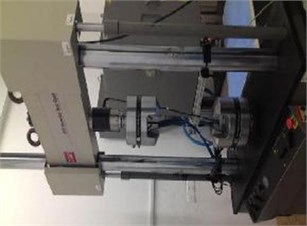Fig. 3MFL-4032 MFL/MMM detector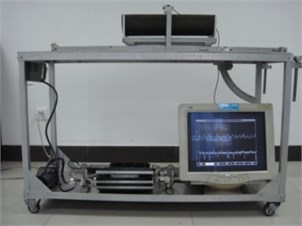Fig. 4Specimen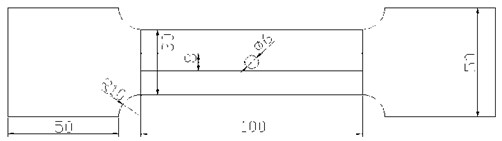### 4.2. Experimental process

During the experiment, in order to avoid the interference of metal clamping device on the signal, a non-magnetic clamping device was used to clamp the magnetic memory detector probe of mfl-4032 magnetic flux leakage / magnetic memory detector. The magnetic memory testing probe is perpendicular to the specimen to be tested, and the lifting height of the magnetic memory testing probe is 0.5 mm. The clamping device advances along the detection line as shown in Fig. 2 at a fixed speed. The magnetic memory signal on the surface of the specimen in the initial state was measured. When the tensile stress of the specimen reaches 220 MPa, 235 MPa and 460 MPa respectively, the magnetic memory signal on the surface of the specimen is collected. In order to improve the reliability and accuracy of the test data, each group of data were repeated for 10 times, and the average value was taken as the final result of the group of data (as shown in Fig. 5).

Fig. 5MMMT signals measured under tension load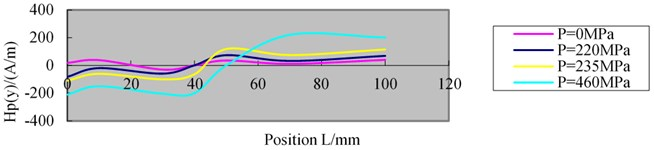### 4.3. Analysis of experimental results

The acquired magnetic memory signal shown in Fig. 5 is decomposed by wavelet packet to analyze its wavelet packet energy spectrum. When the wavelet decomposition level is 3 and the wavelet base is DB2 wavelet, the ideal decomposition effect can be achieved. The magnetic memory signals under different tensile stress are decomposed by three-layer wavelet packet, which produces eight orthogonal wavelet spaces. At this time, the signal is divided into eight non coincident sub bands, of which 1~4 bands are the low frequency band and 5~8 bands are the high frequency band. The wavelet packet energy spectrum of the magnetic memory signal is normalized, and the wavelet packet energy spectrum of the magnetic memory signal is expressed in the form of histogram, as shown in Fig. 6-8.

Fig. 6Energy spectrum of magnetic memory wavelet packet under tensile stress of 220 MPa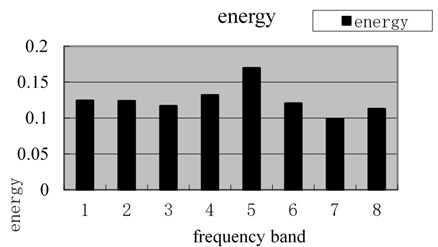Fig. 7Energy spectrum of magnetic memory wavelet packet under tensile stress of 235 MPa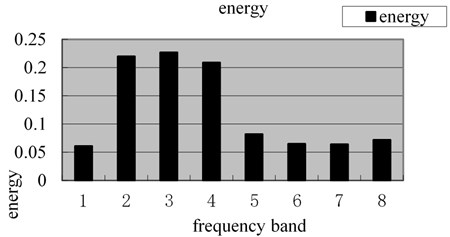Fig. 8Energy spectrum of magnetic memory wavelet packet under tensile stress of 460 MPa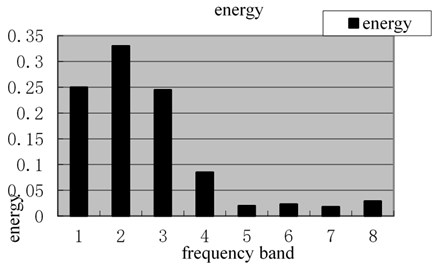It can be seen from Fig. 6-8 that when the tensile stress of the specimen is 220 MPa, the specimen is in the elastic strain stage. Therefore, the stress concentration of the whole specimen is low. At this time, the wavelet packet energy spectrum distribution of the magnetic memory signal is relatively uniform, and the percentage of wavelet packet energy in the total energy of each frequency band is less than 17 %. When the tensile stress of the specimen increases to 235 MPa, the larger value of signal wavelet packet energy is distributed in 2, 3 and 4 frequency bands. The sum of wavelet packet energy in 2, 3 and 4 frequency bands accounts for 65.6 % of the total energy, so the wavelet packet energy spectrum is mainly concentrated in the low frequency band. When the specimen yields, the wavelet packet energy spectrum of the magnetic memory signal is mainly distributed in 1-3 frequency bands. The sum of wavelet packet energy in 1-3 frequency band accounts for 82.5 % of the total energy. Therefore, the energy spectrum distribution is very concentrated, and the main energy is concentrated in the low frequency band.

It can be seen from the experimental results that the higher the stress concentration is, the more concentrated the distribution of energy spectrum is, and it is highly concentrated to the low frequency band. After three-layer wavelet packet decomposition, the frequency band energy information can represent the stress concentration information of the specimen. The magnitude and distribution characteristics of the spectrum energy of each frequency band of magnetic memory signal are closely related to the degree of stress concentration.

## 5. Conclusions

The magnitude and distribution characteristics of the spectrum energy of each frequency band of magnetic memory signal are closely related to the degree of stress concentration. The higher the stress concentration, the more concentrated the energy spectrum distribution, and to the low frequency. On the contrary, the more scattered the energy spectrum is. Therefore, the wavelet packet energy spectrum of magnetic memory signal is a new signal feature which can be used for quantitative identification of stress concentration.